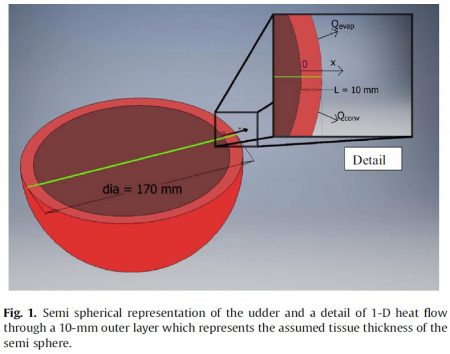## Modelling heat loss from a semi-spherical cow udder

Theoretical physicists are sometimes accused of making over-simplifications for mathematical models. This has lead to many variations on the spherical cow story, where a physicist claims to be able to cure a sick cow, but only if it is a spherical cow in a vacuum. Oddly, most iterations of this tale ignore heat radiated from a cow.

In a classic case of life semi imitating art, researchers Kifle Gebremedhin and Binxin Wu focus on heat radiated from only one part of a dairy cow in the paper titled “Modelling heat loss from the udder of a dairy cow.” Since they are using a semi cow, they decided to use a semi sphere to model the udder.According to the researchers, it only takes ten assumptions to reduce a fully functioning, mooing, milk producing cow into a semi spherical udder model:

1. Cow udder is semi sphere.
2. Radius of the semi sphere is 0.17 m.
3. An outer layer of 10-mm thickness of tissue was assumed for
heat transfer between the udder and ambient air (Fig. 1).
4. A one-dimensional transient (a function of time) heat flow was
assumed through the thickness of the tissue (Fig. 1).
5. Heat lost from the udder skin surface when the cow is standing is by evaporation and convection.
6. Core temperature of udder tissue is 38.6°C.
7. Metabolic heat production rate in the tissue is 1946 W/m3.
8. Blood-flow time through the udder takes between 2 and 4 min.
9. The physical and thermal properties are assumed to be constant.
10.Temperature of blood entering the udder is equal to core temperature.

As noted here before, other models for determining heat loss from whole cows have been around for some time. Some models treated cows as cylinders, while others ignored cow geometry completely and used temperatures and humidity. This particular model is unique in that it simplifies the the cow to a single organ. But how was that organ selected?

Dairy cows are usually cooled off by spraying water from above to wet their bodies. Gebremedhin tried the inverted tactic of wetting the cows from below in “Alternative Cooling of Dairy Cows by udder Wetting,Thermal images of an udder before and after wetting.

Cooling  the udder was just as effective as trying to cool off the entire body of the cow. Gebremedhin found that the massive amount of blood flowing through the udder likely distributes the cooling quickly.

The semi-spherical udder model paper shares the result:

… the udder could be considered as a heat sink, and thus, in time of heat stress, a dairy cow could be cooled by cooling the udder…